# Test: Frequency Domain Sampling

## 10 Questions MCQ Test Digital Signal Processing | Test: Frequency Domain Sampling

Description
Attempt Test: Frequency Domain Sampling | 10 questions in 10 minutes | Mock test for Electrical Engineering (EE) preparation | Free important questions MCQ to study Digital Signal Processing for Electrical Engineering (EE) Exam | Download free PDF with solutions
QUESTION: 1

### If x(n) is a finite duration sequence of length L, then the discrete Fourier transform X(k) of x(n) is given as:

Solution:

Explanation: If x(n) is a finite duration sequence of length L, then the Fourier transform of x(n) is given as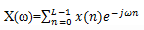If we sample X(ω) at equally spaced frequencies ω=2πk/N, k=0,1,2…N-1 where N>L, the resultant samples are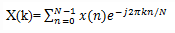QUESTION: 2

### If X(k) discrete Fourier transform of x(n), then the inverse discrete Fourier transform of X(k) is:

Solution:

Explanation: If X(k) discrete Fourier transform of x(n), then the inverse discrete Fourier transform of X(k) is given as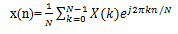QUESTION: 3

### A finite duration sequence of length L is given as x(n) =1 for 0≤n≤L-1=0 otherwise , then what is the N point DFT of this sequence for N=L?

Solution:

Explanation: The Fourier transform of this sequence is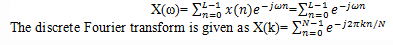If N=L, then X(k)= L for k=0
=0 for k=1,2….L-1

QUESTION: 4

The Nth rot of unity WN is given as:

Solution:

Explanation: We know that the Discrete Fourier transform of a signal x(n) is given as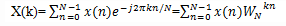Thus we get Nth rot of unity WN= e-j2π/N

QUESTION: 5

Which of the following is true regarding the number of computations requires to compute an N-point DFT?

Solution:

Explanation: The formula for calculating N point DFT is given as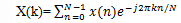From the formula given at every step of computing we are performing N complex multiplications and N-1 complex additions. So, in a total to perform N-point DFT we perform N2 complex multiplications and N(N-1) complex additions.

QUESTION: 6

Which of the following is true?

Solution:

Explanation: If XN represents the N point DFT of the sequence xN in the matrix form, then we know that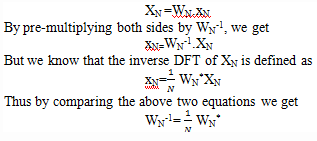QUESTION: 7

What is the DFT of the four point sequence x(n)={0,1,2,3}?

Solution:

Explanation: The first step is to determine the matrix W4. By exploiting the periodicity property of W4 and the symmetry property
WNk+N/2= -WNk
The matrix W4 may be expressed as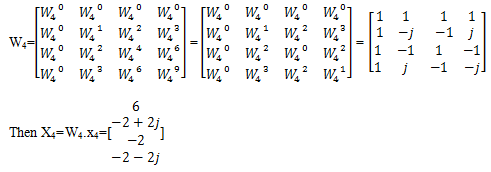QUESTION: 8

If X(k) is the N point DFT of a sequence whose Fourier series coefficients is given by ck, then which of the following is true?

Solution:

Explanation: The Fourier series coefficients are given by the expression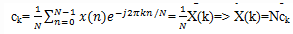QUESTION: 9

What is the DFT of the four point sequence x(n)={0,1,2,3}?

Solution:

Given x(n)={0,1,2,3}
We know that the 4-point DFT of the above given sequence is given by the expression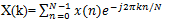In this case N=4
=>X(0)=6,X(1)=-2+2j,X(2)=-2,X(3)=-2-2j.

QUESTION: 10

If W4100=Wx200, then what is the value of x?

Solution:

Explanation: We know that according to the periodicity and symmetry property,
100/4=200/x=>x=8.Use Code STAYHOME200 and get INR 200 additional OFF Use Coupon Code